# Construct a PDA (pushdown automata) for the following language L={0^n 1^m 2^m 3^n | n>=1, m>=1}

Construct a PDA (pushdown automata) for the following language

L={0^n 1^m 2^m 3^n | n>=1, m>=1}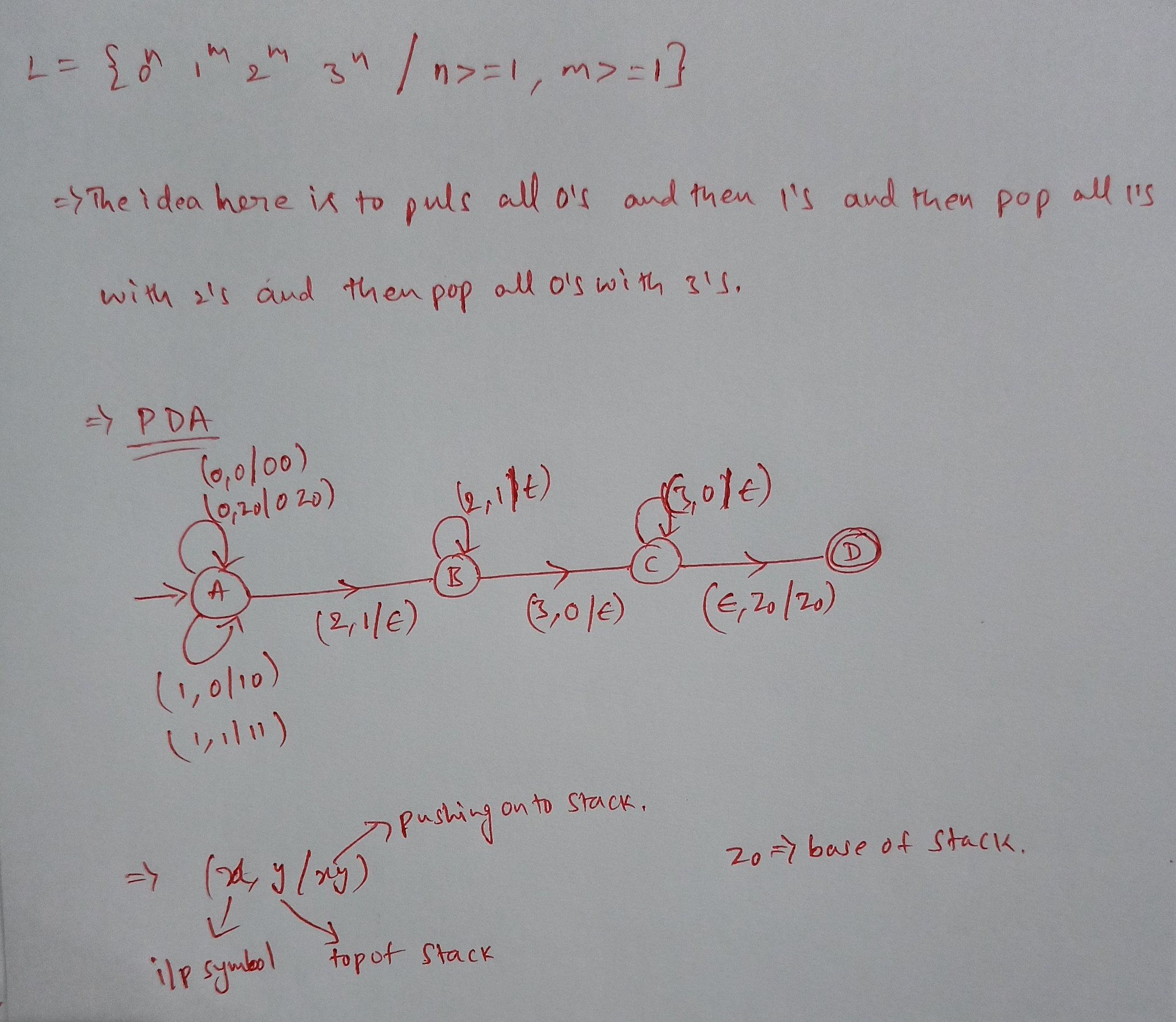##### Add Answer of: Construct a PDA (pushdown automata) for the following language L={0^n 1^m 2^m 3^n | n>=1, m>=1}
Similar Homework Help Questions
• ### 2. [10 marks] Give a PDA (Pushdown Automata) that recognizes the language L = {o€ {n,y,...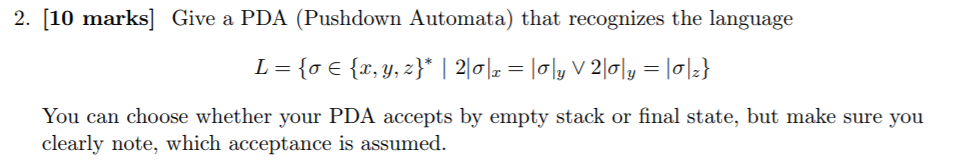2. [10 marks] Give a PDA (Pushdown Automata) that recognizes the language L = {o€ {n,y, z}* | 2|이|z = |0ly V 2\이 You can choose whether your PDA accepts by empty stack or final state, but make sure you clearly note, which acceptance is assumed 2. [10 marks] Give a PDA (Pushdown Automata) that recognizes the language L = {o€ {n,y, z}* | 2|이|z = |0ly V 2\이 You can choose whether your PDA accepts by empty stack or...

• ### A B-bounded PDA (pushdown automaton) is a PDA M such that it crashes whenever its stack...

A B-bounded PDA (pushdown automaton) is a PDA M such that it crashes whenever its stack height reaches B. Show that the language {0n1n : n ≥ 1} can not be accepted by a B-bounded PDA for any B.

• ### Q.3 Maximum score 20 Construct a Non-deterministic PDA that accepts the language L (w: n(w)+n(w) ...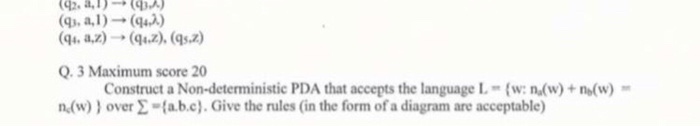answer question 3 Q.3 Maximum score 20 Construct a Non-deterministic PDA that accepts the language L (w: n(w)+n(w) n(w) 1 over 2-(a.b.c).Give the rules (in the form of a diagram are acceptable Q.3 Maximum score 20 Construct a Non-deterministic PDA that accepts the language L (w: n(w)+n(w) n(w) 1 over 2-(a.b.c).Give the rules (in the form of a diagram are acceptable

• ### 1) Given language L = {a"62"n >0} a) Give an informal english description of a PDA...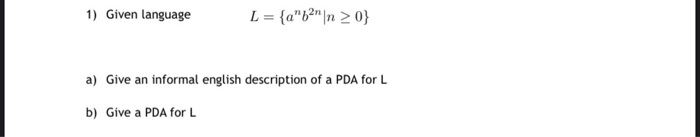1) Given language L = {a"62"n >0} a) Give an informal english description of a PDA for L b) Give a PDA for L

• ### Construct a turning machine for the following language: L = {0^n 1^n 2^n | n >=...

Construct a turning machine for the following language: L = {0^n 1^n 2^n | n >= 1}

• ### Give deterministic pushdown automata to accept the following languages. a) {0n1m | n<=m}. b) {0n1m |...

Give deterministic pushdown automata to accept the following languages. a) {0n1m | n<=m}. b) {0n1m | n>=m}. c) {0n1m0n | n and m are arbitraty}.

• ### Construct a Pushdown automaton that accepts the strings on alphabet {a,b,(, ) }, where parenthesis “(””)”...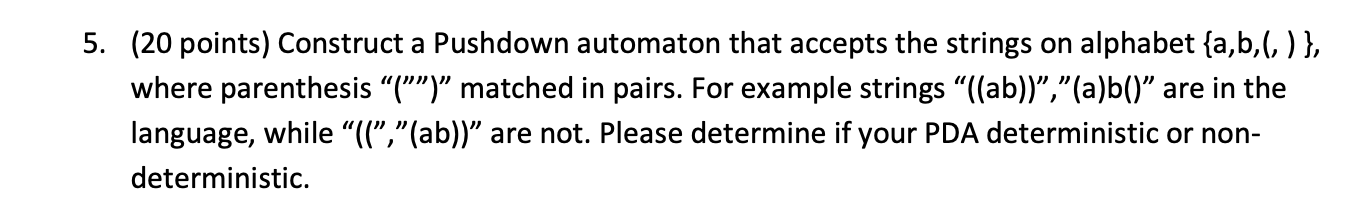Construct a Pushdown automaton that accepts the strings on alphabet {a,b,(, ) }, where parenthesis “(””)” matched in pairs. For example strings “((ab))”,”(a)b()” are in the language, while “((”,”(ab))” are not. Please determine if your PDA deterministic or nondeterministic 5. (20 points) Construct a Pushdown automaton that accepts the strings on alphabet {a,b, (, ) }, where parenthesis “("")" matched in pairs. For example strings "((ab))","(a)b()” are in the language, while “l(","(ab))” are not. Please determine if your PDA deterministic...

• ### (9 pts 3 pts each) For each of the following languages, name the least powerful type of machine that will accept it, an...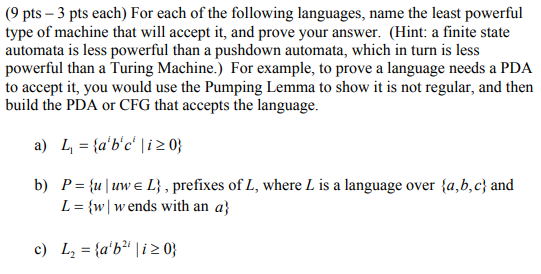(9 pts 3 pts each) For each of the following languages, name the least powerful type of machine that will accept it, and prove your answer. (Hint: a finite state automata is less powerful than a pushdown automata, which in turn is less powerful than a Turing Machine.) For example, to prove a language needs a PDA to accept it, you would use the Pumping Lemma to show it is not regular, and then build the PDA or CFG that...

• ### Let INFINITE PDA ={<M>|M is a PDA and L(M) is an infinite language} Show that INFINITE PDA is decidable

Let INFINITE PDA ={<M>|M is a PDA and L(M) is an infinite language} Show that INFINITE PDA is decidable.

• ### (9 pts 3 pts each) For each of the following languages, name the least powerful type of machine that will accept it...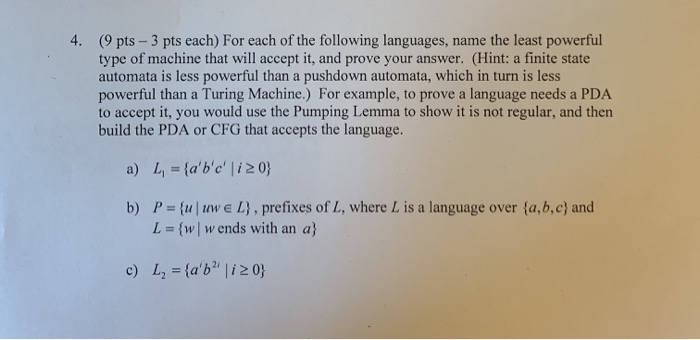(9 pts 3 pts each) For each of the following languages, name the least powerful type of machine that will accept it, and prove your answer. (Hint: a finite state automata is less powerful than a pushdown automata, which in turn is less powerful than a Turing Machine.) For example, to prove a language needs a PDA to accept it, you would use the Pumping Lemma to show it is not regular, and then build the PDA or CFG that...

Free Homework App